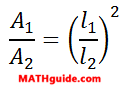Similar Figures and Solids
 Home > Lessons > Similar Figures and Solids Search | Updated December 22nd, 2018
Introduction

In this section of MATHguide, you will learn about the relation between lengths, areas, and volumes of similar figures/solids. Here are the topics within this page:

 Similar Figures: Lengths and Areas This lesson will inform you of the relation between lengths and areas of similar figures. Here is the equation that quantifies the relation.View the lesson that will explain how to use this equation.esson: Areas and Lengths of Similar Figures Similar Solids: Lengths and Volumes This lesson will inform you of the relation between lengths and volumes of similar solids. Here is the equation that quantifies the relation.View the lesson that will explain how to use this equation.esson: Volumes and Lengths of Similar Solids